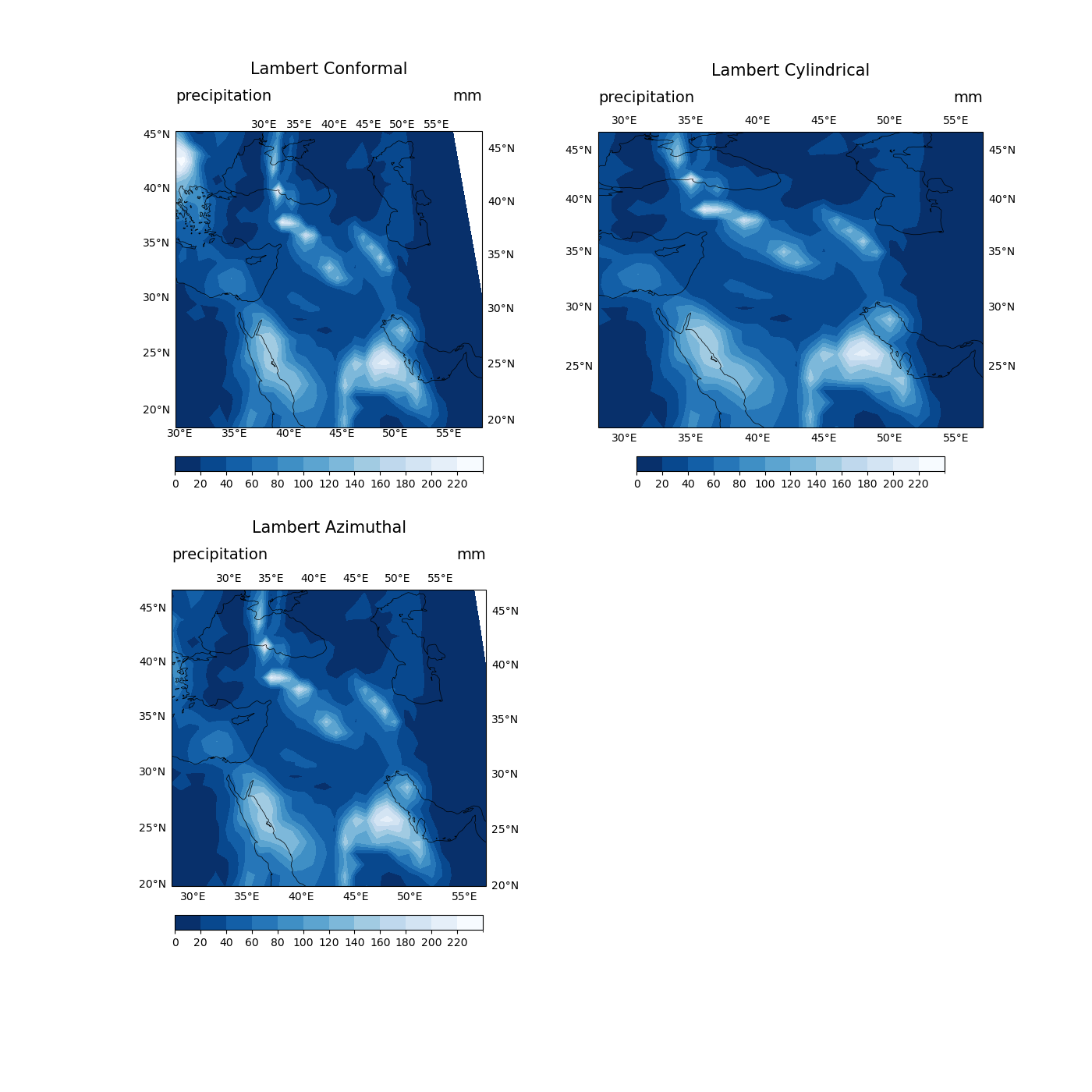# NCL_lcnative_1.py#

This script illustrates the following concepts:
• Drawing contours over a map using a native lat,lon grid

• Drawing filled contours over a Lambert Conformal map

• Zooming in on a particular area on a Lambert Conformal map

• Subsetting a color map

• Using all three Cartopy Lambert projections to find the best fit for visualization

• Implementing best practices for choosing contour color scheme. The original NCL version uses a rainbow color map which can create problems for individuals impacted by color blindness and is not black and white printer friendly. To overcome this, we used the “Blues_r” color scheme from Matplotlib. More information on best practices can be found here.

See following URLs to see the reproduced NCL plot & script:

Import packages:

```import numpy as np
import xarray as xr
import cartopy.crs as ccrs
import matplotlib.pyplot as plt
import matplotlib.ticker as mticker

import geocat.datafiles as gdf
```

```# Open a netCDF data file using xarray default engine and load the data into xarrays
ds = xr.open_dataset(gdf.get("netcdf_files/pre.8912.mon.nc"),
decode_times=False)

# Extract a slice of the data
t = ds.pre.isel(time=0)
```

Plot:

```plt.figure(figsize=(14, 14))

def Plot(row, col, pos, proj, title):
'''
Args:

row (:class: 'int'):
number of rows necessary for subplotting of visualizations
col (:class: 'int'):
number of columns necessary for subplotting
pos (:class: 'int'):
position of visualization in m x n subplot
proj (:class: 'cartopy.crs'):
which projection to visualize
title (:class: 'str'):
center title of respective visualization
'''
# Generate axes using Cartopy and draw coastlines
ax = plt.subplot(row, col, pos, projection=proj)
ax.set_extent((28, 57, 20, 47), crs=ccrs.PlateCarree())
ax.coastlines(linewidth=0.5)

# Use ax "gridlines" function to draw lat/lon markers on projections
gl = ax.gridlines(draw_labels=True,
dms=False,
x_inline=False,
y_inline=False)
gl.top_labels = True
gl.right_labels = True
gl.xlines = False
gl.ylines = False
gl.xlocator = mticker.FixedLocator([30, 35, 40, 45, 50, 55])
gl.ylocator = mticker.FixedLocator([20, 25, 30, 35, 40, 45])
gl.xlabel_style = {'rotation': 0}
gl.ylabel_style = {'rotation': 0}
'''
When using certain types of projections in Cartopy, you may find that there
is not a direct 1-to-1 projection similarity. When looking at the three Lambert
projections offered, you will notice the closest match to the NCL projection
is actually the Lambert Cylindrical projection. This is due to NCL having certain
"smoothing" and "flattening" options for the Lambert Conformal projection not seen
in the Cartopy version. By using Lambert Cylindrical over Lambert Conformal in Python,
you will be able to create the "rectangular" style of coordinates not classically
represented by a Lambert Conformal map. Additionally, Cartopy does not currently support
adding tick marks to a projection like NCL, this is why these Python projections do not
have this feature. The GeoCAT Team is actively adding to the list of convenience functions
supported and hopes to add this functionality one day.
'''

# Plot data and create colorbar
prec = t.plot.contourf(ax=ax,
cmap="Blues_r",
transform=ccrs.PlateCarree(),
levels=14,

cbar_ticks = np.arange(0, 240, 20)
cbar = plt.colorbar(prec,
orientation='horizontal',
shrink=0.8,
ticks=cbar_ticks)

cbar.ax.tick_params(labelsize=10)
plt.title(title, loc='center', y=1.17, size=15)
plt.title(t.units, loc='right', y=1.08, size=14)
plt.title("precipitation", loc='left', y=1.08, size=14)

Plot(
2, 2, 1,
ccrs.LambertConformal(central_longitude=45,
standard_parallels=(36, 55),
globe=ccrs.Globe()), "Lambert Conformal")
Plot(2, 2, 2, ccrs.LambertCylindrical(central_longitude=45),
"Lambert Cylindrical")
Plot(2, 2, 3, ccrs.LambertAzimuthalEqualArea(central_longitude=45),
"Lambert Azimuthal")
```Total running time of the script: ( 0 minutes 2.244 seconds)

Gallery generated by Sphinx-Gallery